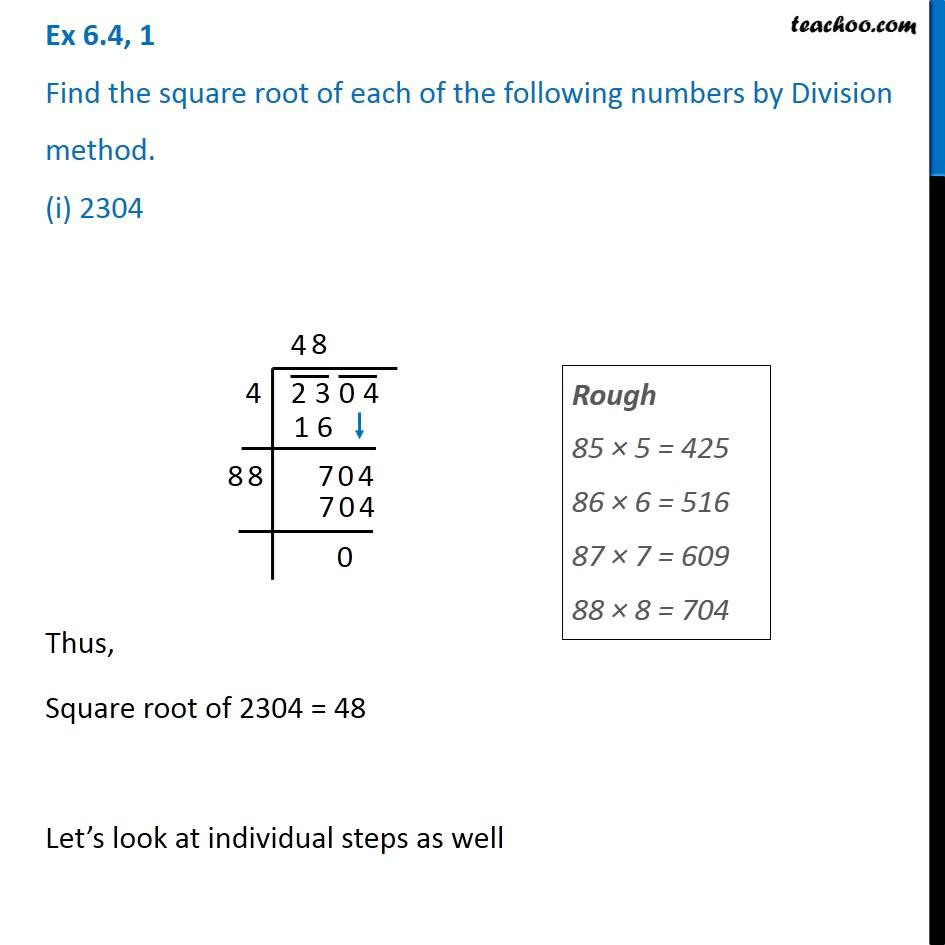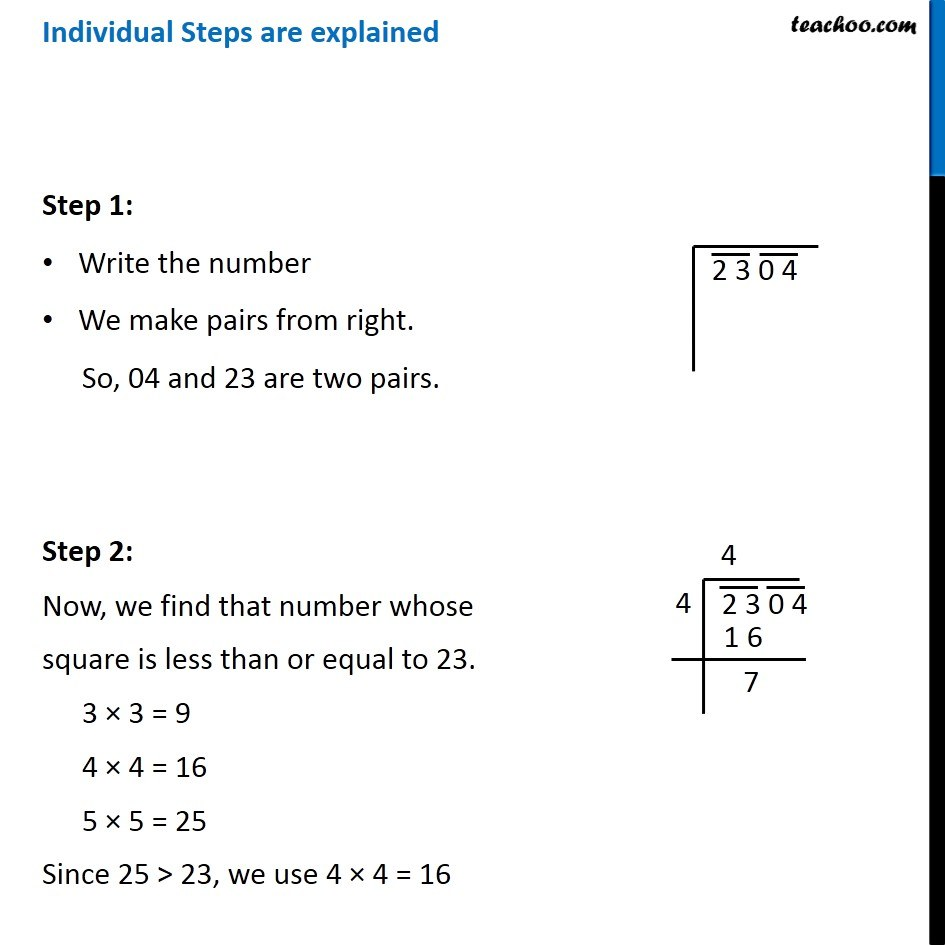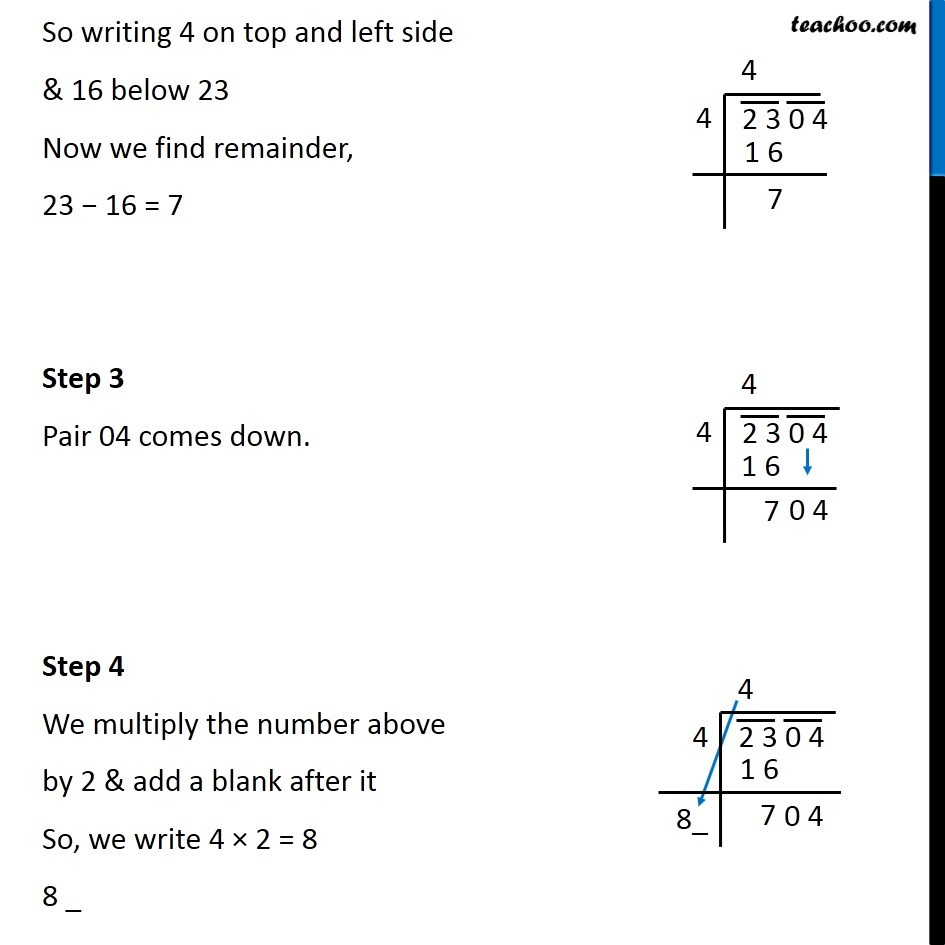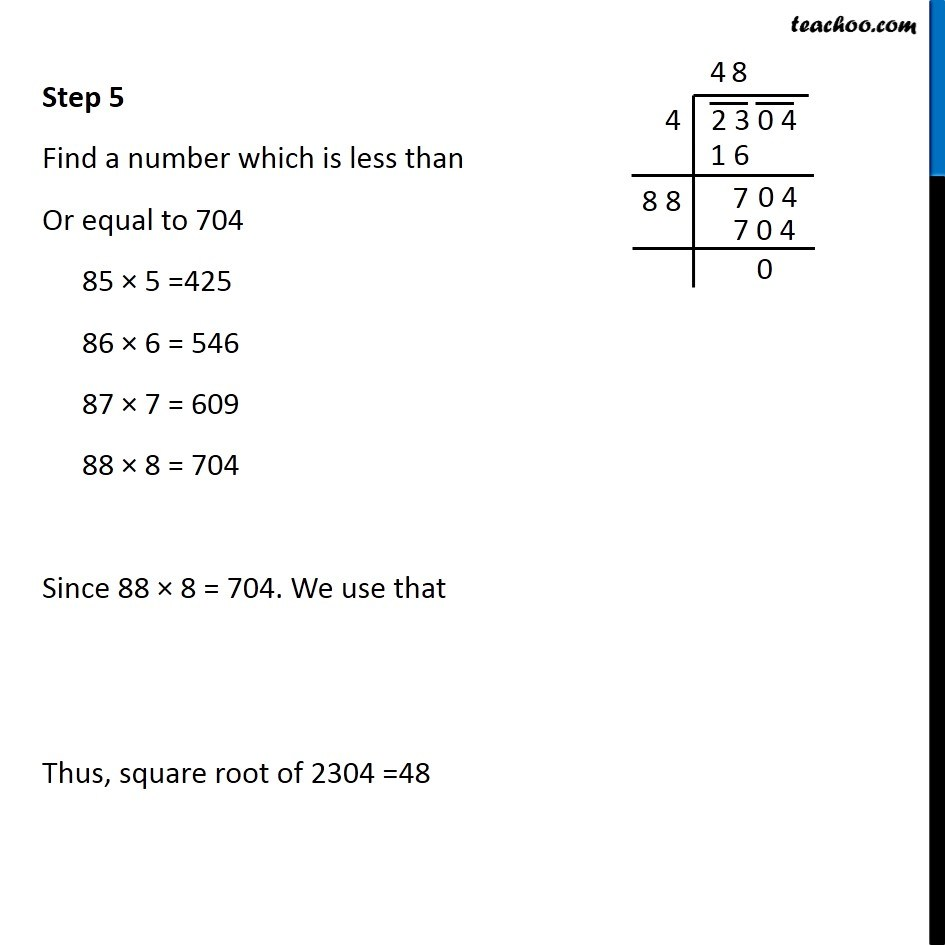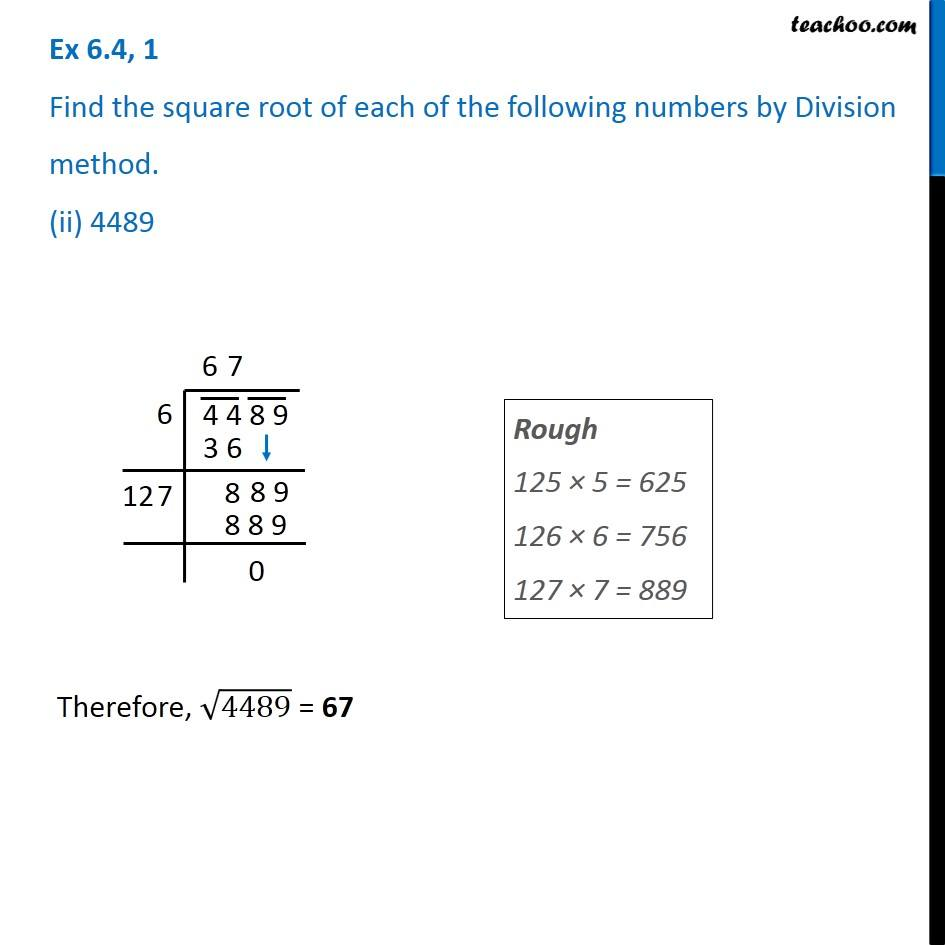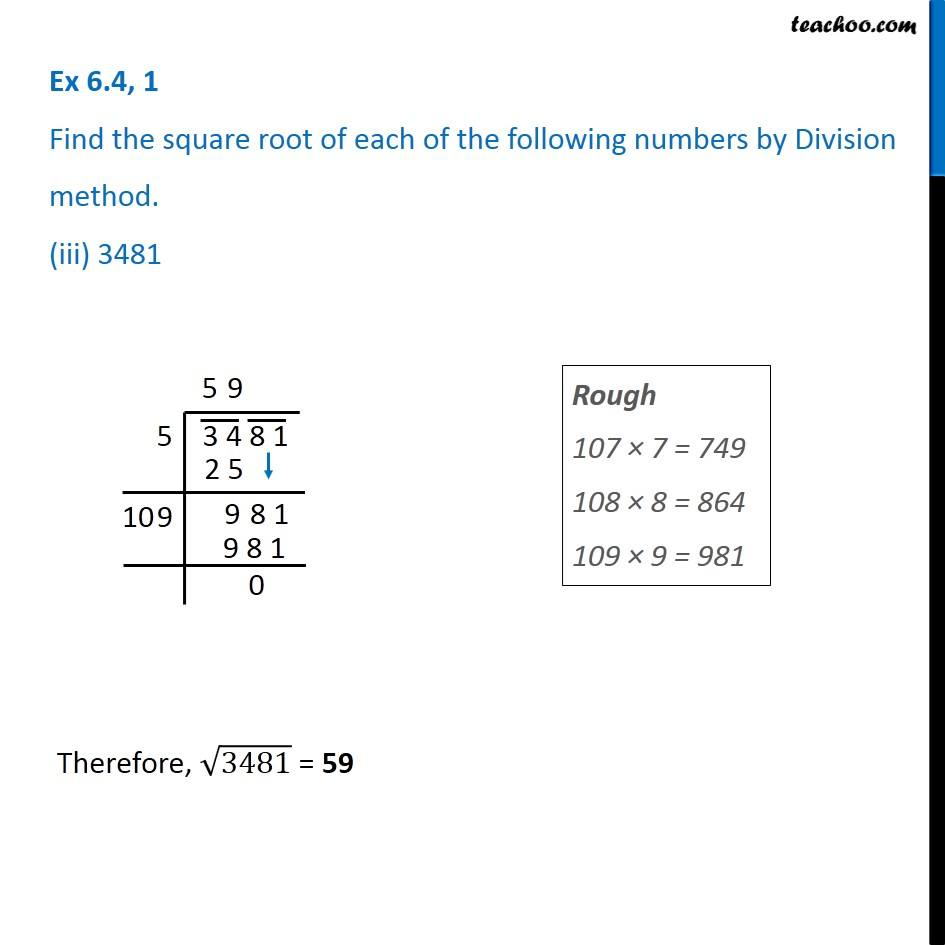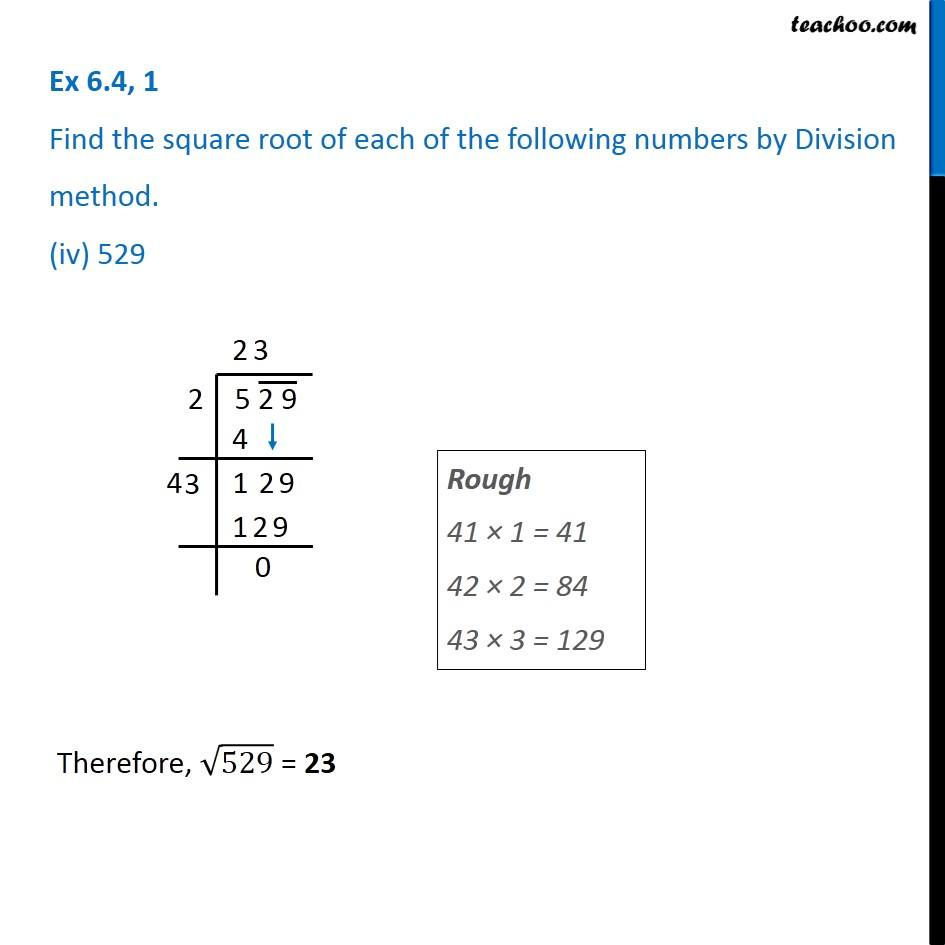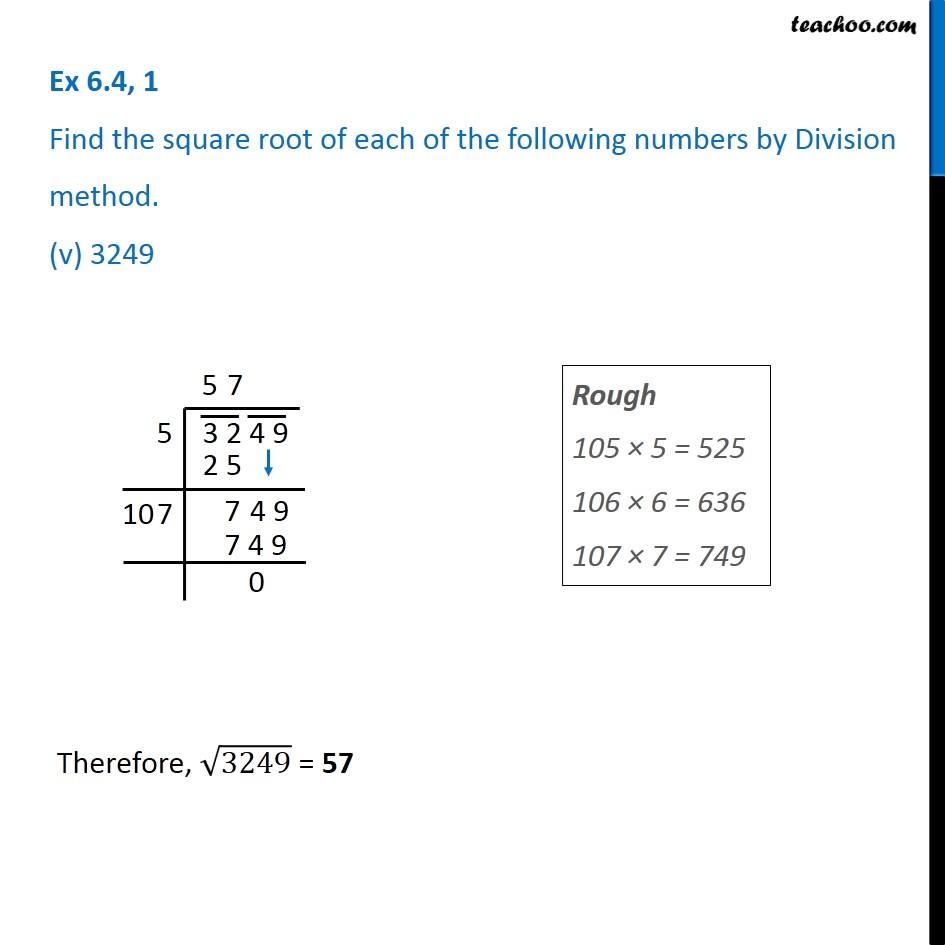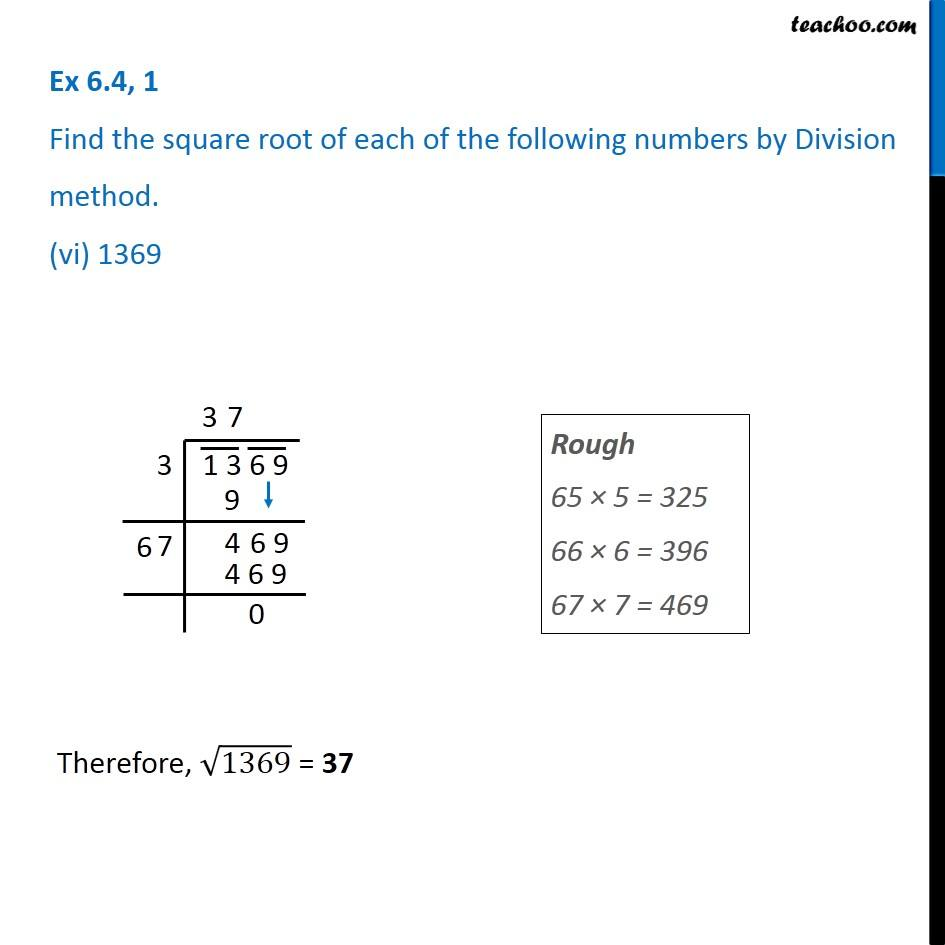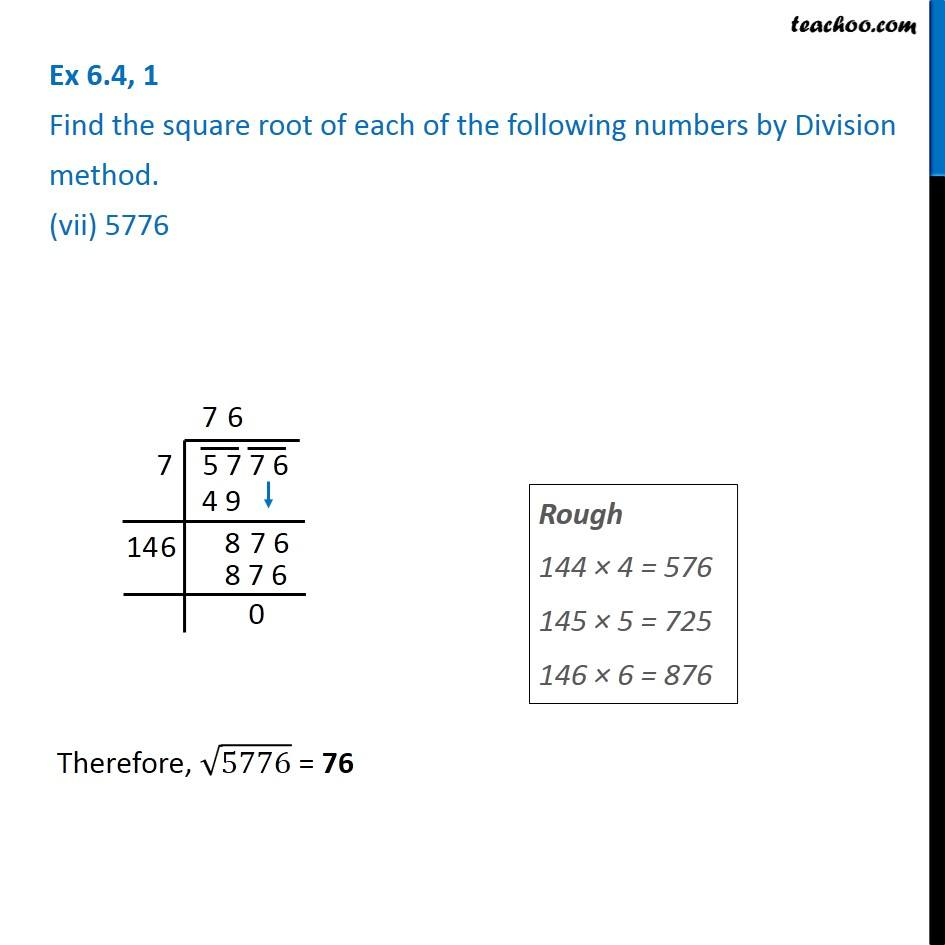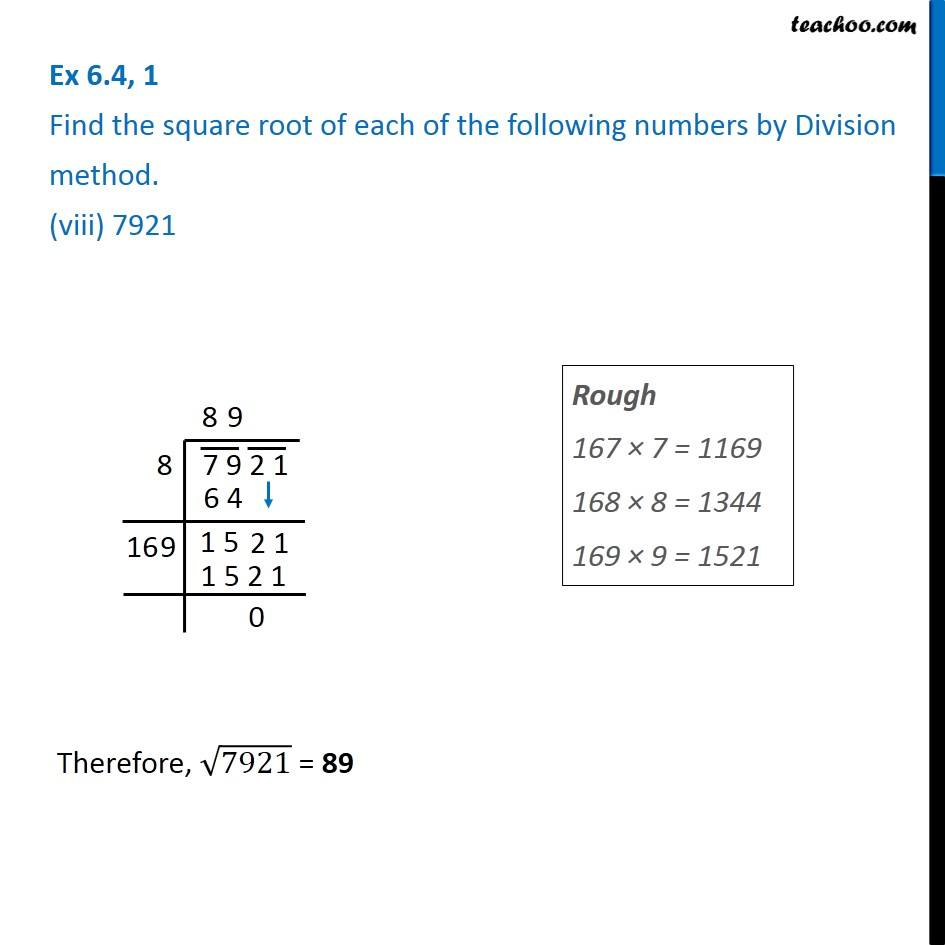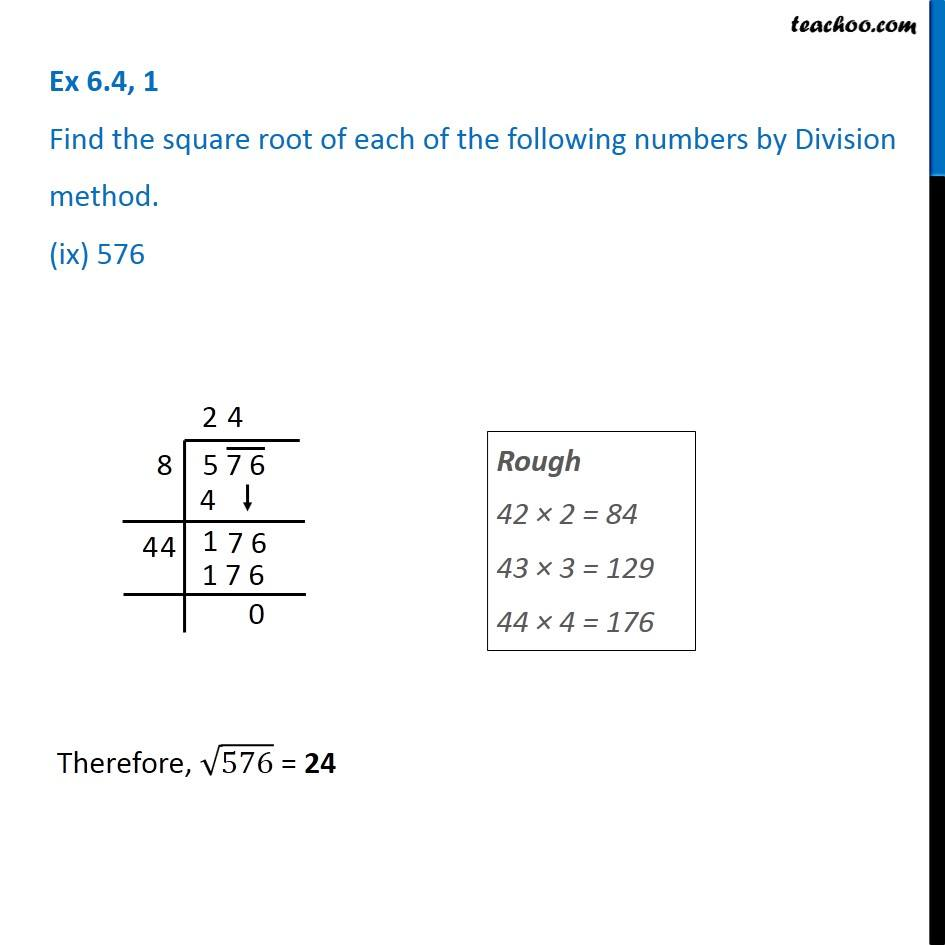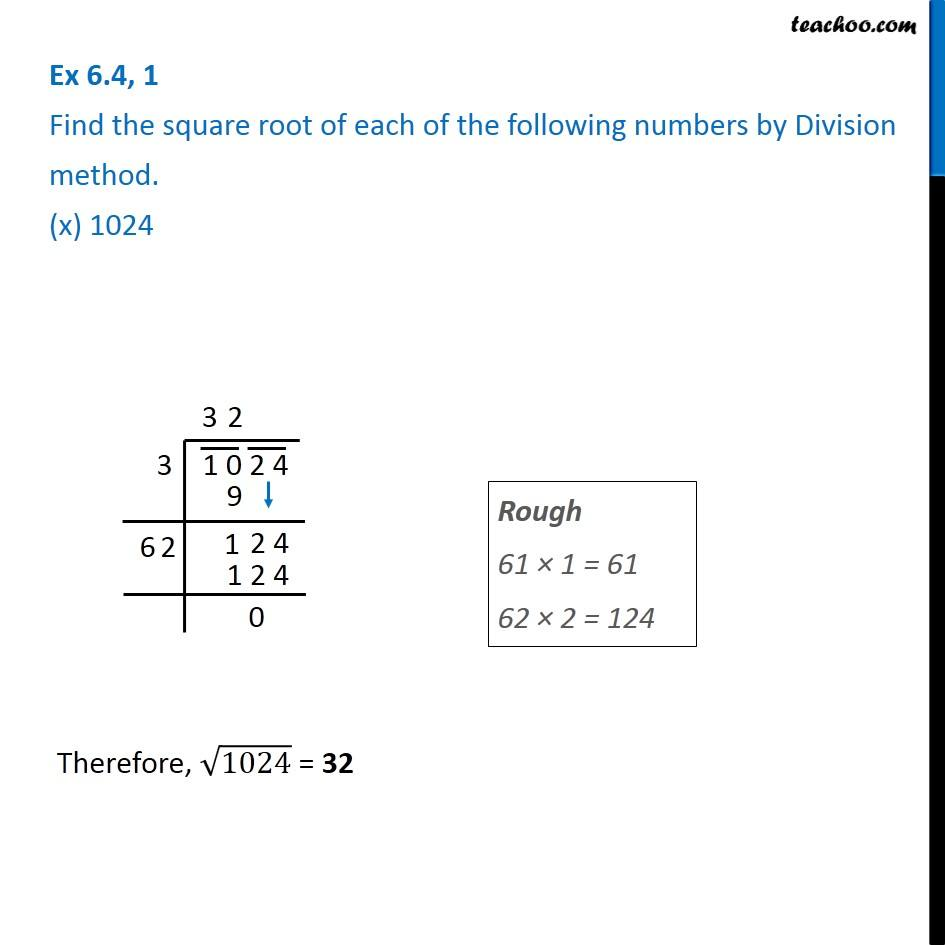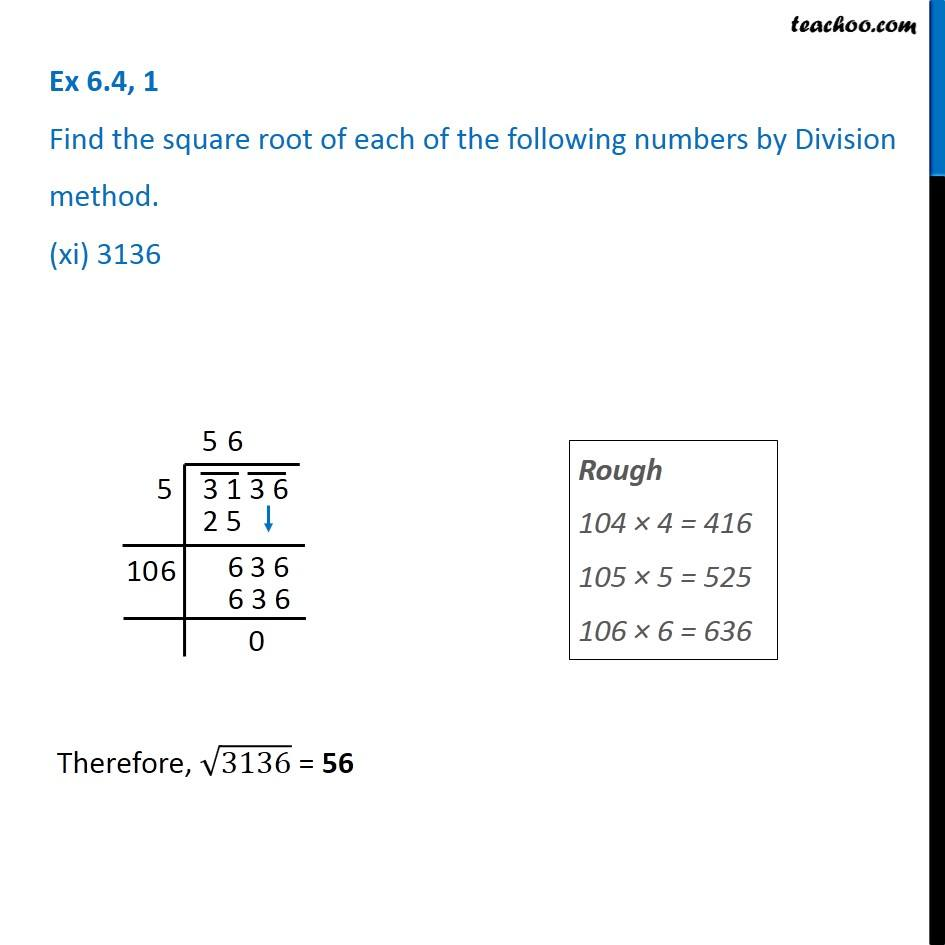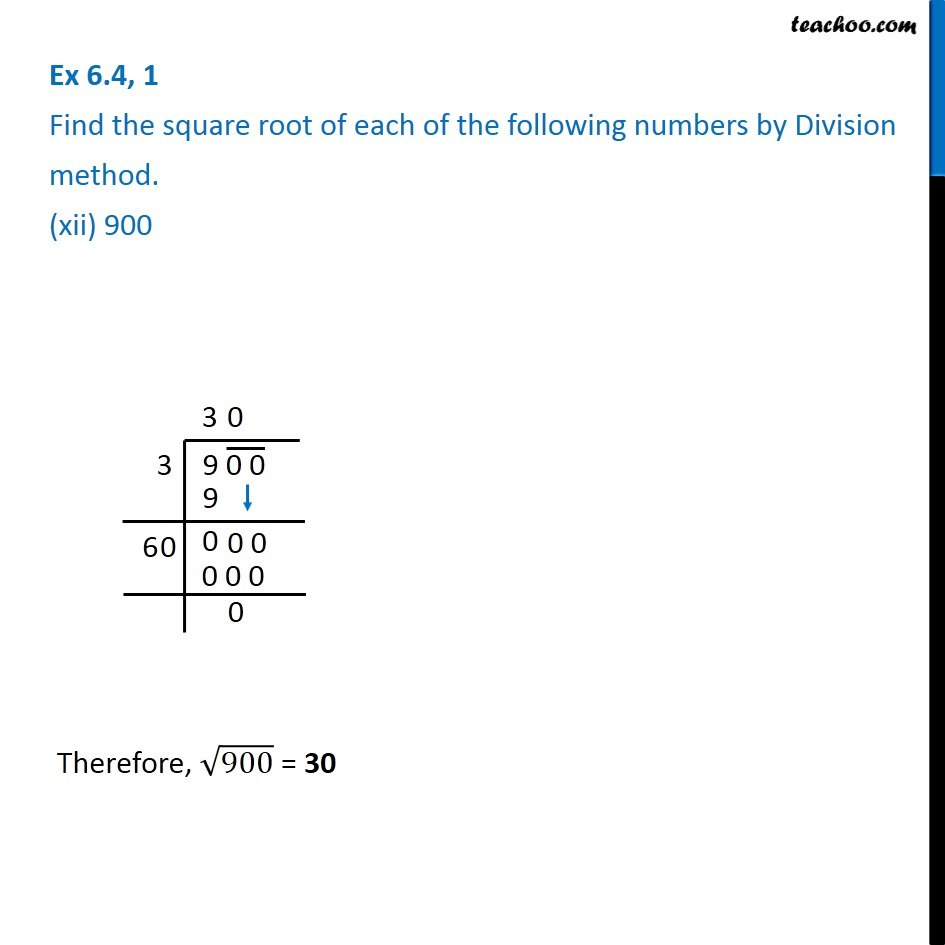Subscribe to our Youtube Channel - https://you.tube/teachoo

1. Chapter 6 Class 8 Squares and Square Roots
2. Serial order wise
3. Ex 6.4

Transcript

Ex 6.4, 1 Find the square root of each of the following numbers by Division method. (i) 2304 Thus, Square root of 2304 = 48 Let’s look at individual steps as well Individual Steps are explained Step 1: Write the number We make pairs from right. So, 04 and 23 are two pairs. Step 2: Now, we find that number whose square is less than or equal to 23. 3 × 3 = 9 4 × 4 = 16 5 × 5 = 25 Since 25 > 23, we use 4 × 4 = 16 So writing 4 on top and left side & 16 below 23 Now we find remainder, 23 − 16 = 7 Step 3 Pair 04 comes down. Step 4 We multiply the number above by 2 & add a blank after it So, we write 4 × 2 = 8 8 _ Step 5 Find a number which is less than Or equal to 704 85 × 5 =425 86 × 6 = 546 87 × 7 = 609 88 × 8 = 704 Since 88 × 8 = 704. We use that Thus, square root of 2304 =48 Ex 6.4, 1 Find the square root of each of the following numbers by Division method. (ii) 4489 Therefore, √4489 = 67 Ex 6.4, 1 Find the square root of each of the following numbers by Division method. (iii) 3481 Rough 107 × 7 = 749 108 × 8 = 864 109 × 9 = 981 Therefore, √3481 = 59 Ex 6.4, 1 Find the square root of each of the following numbers by Division method. (iv) 529 Rough 41 × 1 = 41 42 × 2 = 84 43 × 3 = 129 Therefore, √529 = 23 Ex 6.4, 1 Find the square root of each of the following numbers by Division method. (v) 3249 Rough 105 × 5 = 525 106 × 6 = 636 107 × 7 = 749 Therefore, √3249 = 57 Ex 6.4, 1 Find the square root of each of the following numbers by Division method. (vi) 1369 Rough 65 × 5 = 325 66 × 6 = 396 67 × 7 = 469 Therefore, √1369 = 37 Ex 6.4, 1 Find the square root of each of the following numbers by Division method. (vii) 5776 Rough 144 × 4 = 576 145 × 5 = 725 146 × 6 = 876 Therefore, √5776 = 76 Ex 6.4, 1 Find the square root of each of the following numbers by Division method. (viii) 7921 Rough 167 × 7 = 1169 168 × 8 = 1344 169 × 9 = 1521 Therefore, √7921 = 89 Ex 6.4, 1 Find the square root of each of the following numbers by Division method. (ix) 576 Rough 42 × 2 = 84 43 × 3 = 129 44 × 4 = 176 Therefore, √576 = 24 Ex 6.4, 1 Find the square root of each of the following numbers by Division method. (x) 1024 Rough 61 × 1 = 61 62 × 2 = 124 Ex 6.4, 1 Find the square root of each of the following numbers by Division method. (xi) 3136 Rough 104 × 4 = 416 105 × 5 = 525 106 × 6 = 636 Therefore, √3136 = 56 Ex 6.4, 1 Find the square root of each of the following numbers by Division method. (xii) 900 Therefore, √900 = 30

Ex 6.4